The K5 Learning Blog urges parents to be pro-active in helping their children reach their full academic potential.

K5 Learning
provides free worksheets, workbooks and an online reading and math program for kindergarten to grade 5 students.Learning to add numbers in your head is an essential part of your child’s math journey. There are a number of methods students can use to make adding numbers using mental math much easier. Here we share some of those addition tips and tricks.

## Friendly numbers

For this technique, imagine a number line. You make a ‘friendly’ number first and then add the rest. Let us show you how.

For example: 17 + 7

Making a friendly number means a number ending in 0.
For 17, that friendly number is 20.
You borrow 3 from 7 to make it 20.
That means you have 4 left: 7 – 3.
Here’s how that looks on a number line: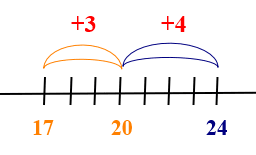## Stealing numbers

At times it’s easier to ‘steal’ a number; let us show you how.

For example: 59 + 86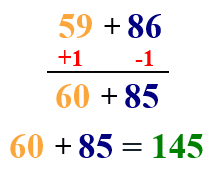## Compensation Method

In compensation you round up a number – to make it easier to add – and then take away the extra after you have added.

For example: 29 + 16

It’s easier to round up the 29 to 30, and then take away the extra 1: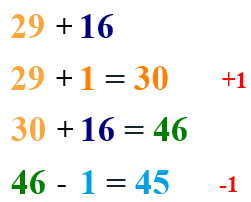## Break apart by place value

Sometimes it may be easier to deal with the numbers by place value: adding the tens together, adding the ones together and adding the total numbers.

For example: 36 + 72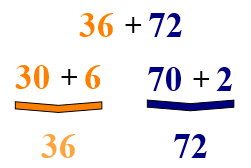Now let’s re-group the numbers into tens and ones. Add the tens and ones separately.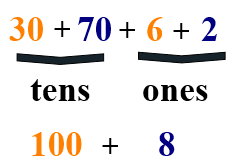Now it’s easier to do the final sum: 100 + 8 = 108

To practice these methods, we have a selection of mental addition worksheets in our grade 4 math section.